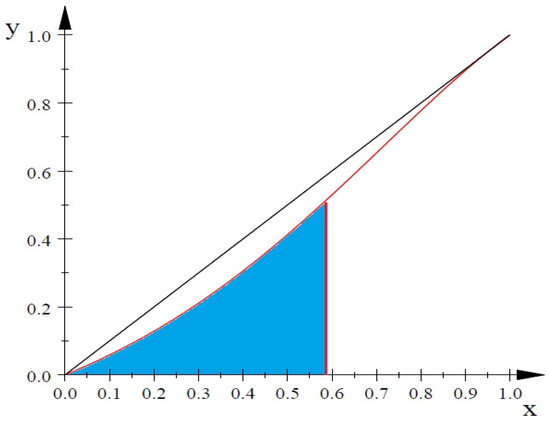Next Article in Journal
A Classification of Symmetric (1, 1)-Coherent Pairs of Linear Functionals
Previous Article in Journal
Stability Analysis of Singly Diagonally Implicit Block Backward Differentiation Formulas for Stiff Ordinary Differential Equations
Previous Article in Special Issue
Ground State Solutions for Fractional Choquard Equations with Potential Vanishing at Infinity

## Volume 7, Issue 2Metrics 0

## Export Article

Open AccessFeature PaperArticle
Mathematics 2019, 7(2), 212; https://doi.org/10.3390/math7020212

# On Positive Quadratic Hyponormality of a Unilateral Weighted Shift with Recursively Generated by Five Weights

1
Department of Mathematics, Northeastern University, Shenyang 110-004, China
2
Department of Mathematics, Hannam University, Daejeon 34430, Korea
*
Author to whom correspondence should be addressed.
Received: 16 January 2019 / Revised: 7 February 2019 / Accepted: 8 February 2019 / Published: 25 February 2019
(This article belongs to the Special Issue Polynomials: Theory and Applications)
|   PDF [314 KB, uploaded 25 February 2019]   |

# Abstract

Let $1 < a < b < c < d$ and $α ^ 5 : = 1 , a , b , c , d ∧$ be a weighted sequence that is recursively generated by five weights $1 , a , b ,$ $c , d$ . In this paper, we give sufficient conditions for the positive quadratic hyponormalities of $W α x$ and $W α y , x$ , with $α x : x , α ^ 5$ and $α y , x : y , x , α ^ 5 .$ View Full-Text
FiguresFigure 1

This is an open access article distributed under the Creative Commons Attribution License which permits unrestricted use, distribution, and reproduction in any medium, provided the original work is properly cited (CC BY 4.0).MDPI and ACS Style

Li, C.; Ryoo, C.S. On Positive Quadratic Hyponormality of a Unilateral Weighted Shift with Recursively Generated by Five Weights. Mathematics 2019, 7, 212.

Note that from the first issue of 2016, MDPI journals use article numbers instead of page numbers. See further details here.

1